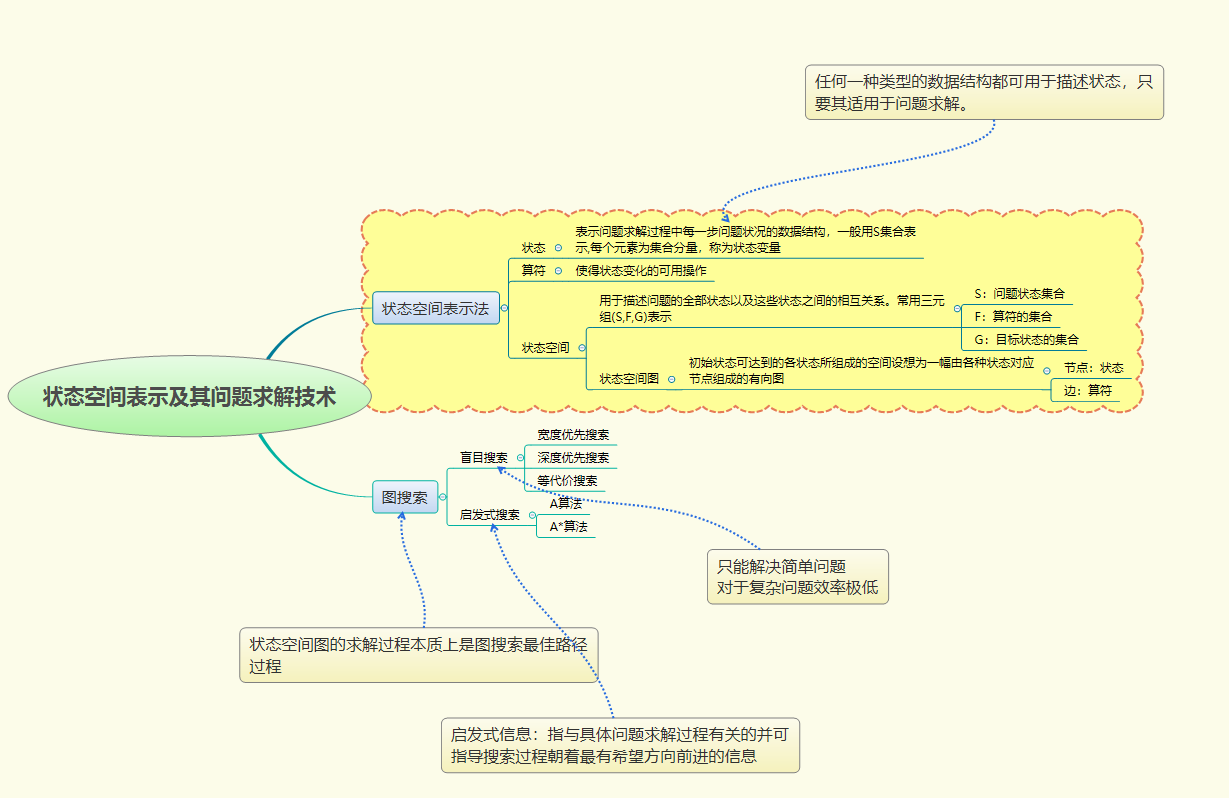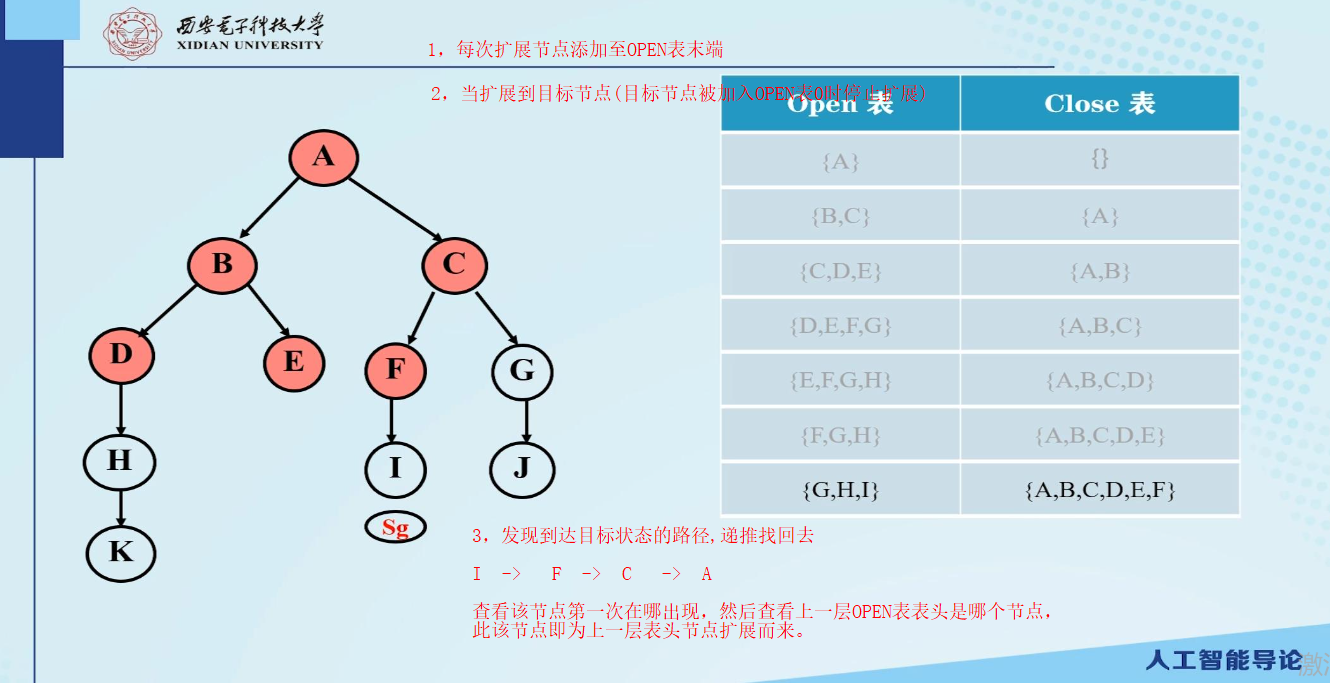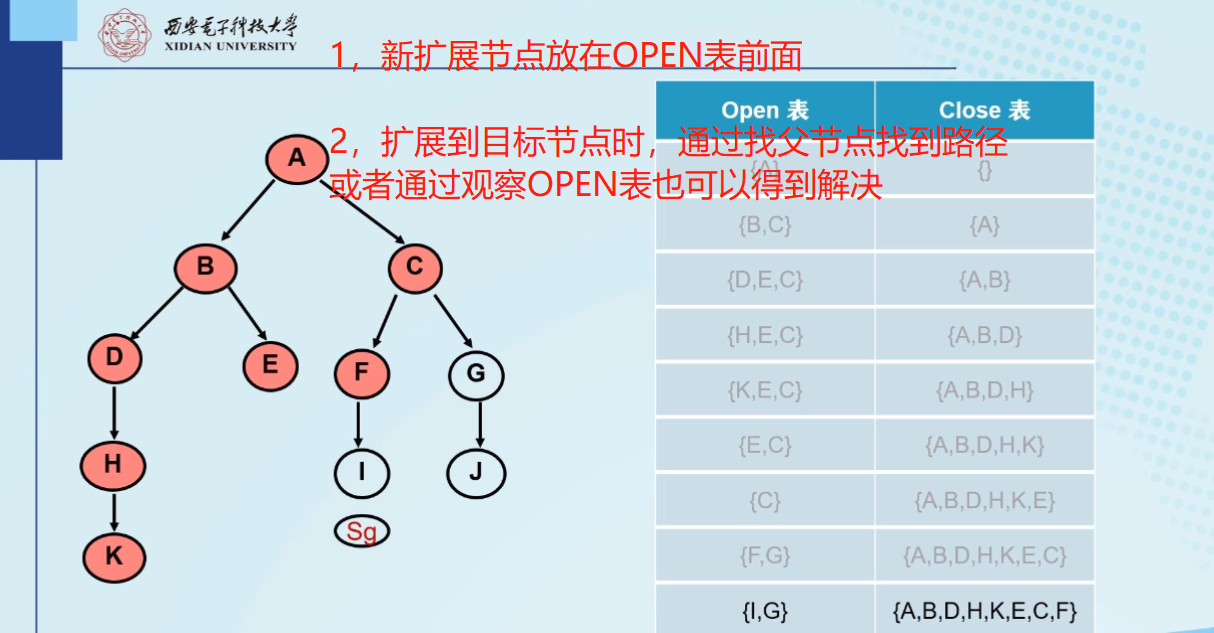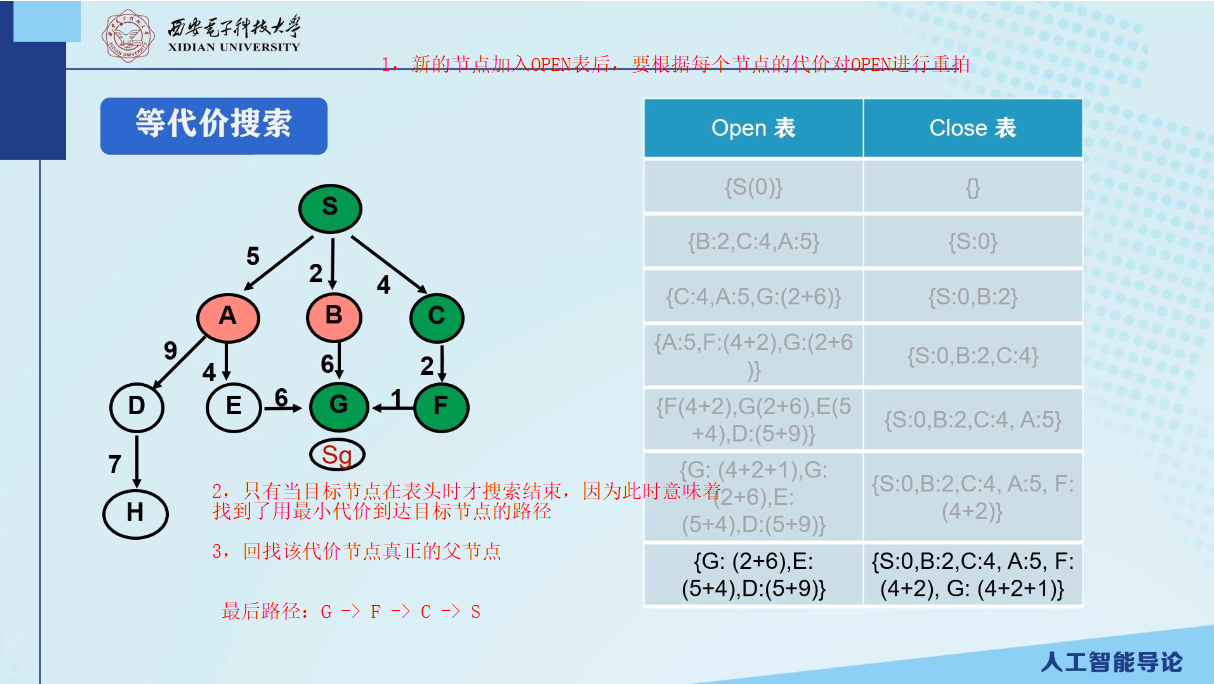# 西安电子科技大学week01-状态空间表示及其问题解决1，盲目搜索

1.1 宽度优先搜索

基本思想：

•  扩展初始节点
• 扩展初始节点的所有后继节点
• 扩展后继节点的后继节点
• 第n层结束，不可能到n+1层

• 新扩展节点放于OPEN表末端
• 问题有解时，一定可以找到
• 方法具有通用性
• 数据规模大时，搜索效率低

1.2 深度优先搜素

• 总是扩展当前节点的最深节点
• 一直扩展直到到达最深层，直至没有下一层
• 回退到上一个还有未扩展后继节点的上层继续扩展

任何其他节点的深度为d=d(父)+1

• 不能保证最优解
• 存在无穷分支时可能不能找到解(此时可以借助深度界限解决，一些问题上极为重要)

1.3 等代价搜索

• g(n)表示从初始节点到当前节点n的代价
• c(n1，n2)表示从父节点n1到其子节点n2的代价
• g(n2) = g(n1) + c(n1，n2)

2，启发式搜索

f(n) = g(n) + h(n)

g(n)表示从起始节点到当前节点的代价

h(n)表示从当前节点到目标节点的代价

2.1 A算法

•  要点：用f(n)算出每个子节点的估价，根据该估价对OPEN表进行重排，只对扩展节点这一部分重排，估价越小排在越前面。

•  要点：用f(n)算出每个子节点的估价，根据该估价对OPEN表进行重排，对OPEN中所有节点进行重排，估价越小排在越前面。

2.2 A*算法

• 背景：A算法不确定能保证找到最优估价函数，A*算法在A算法的基础上添加一点小改动，使得估价函数能够使最佳，从而找到最佳路径、
• f(n) = g(n) + h(n)

在A算法的基础上，g(n)>0 , h(n) 是 h*(n) 的下界，即对于任意n , h(n) < =h*(n) , 在满足h(n) < =h*(n)的条件下，h(n) 越大越好。

h(n) = 0时，则等价为等代价算法。

3，例题理解posted on 2021-01-31 23:03  松岛菜  阅读(193)  评论(0编辑  收藏  举报